Forward Differencing Equations for Second-Order Iteration

Recall how we used forward finite differencing to simulate the behavior of a function that changed proportional to its current size in Lecture 19.  Consider now a function that changes "how fast it changes" proportional to its current change and its current value--in other words its current acceleration is proportional to its velocity and to its size:

Let's work out how to find a finite differencing function:

This is the current change or approximation to velocity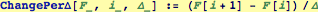This is the current change in change or approximation to acceleration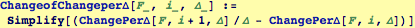Our model is that the acceleration is proportional to  size of the current function and its velocity, let these proportions be: -α and -β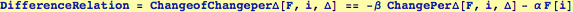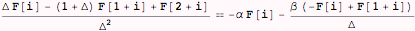Solve this for the "latest" values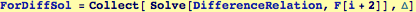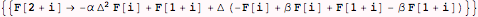Replace to find the form of the solution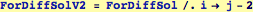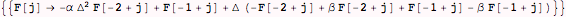Created by Wolfram Mathematica 6.0  (16 November 2007)